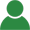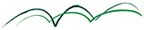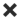ErrorHome Courses Course Calendar Teaching Team About Us Resources Contact
Course Calendar

# Modern Asset Allocation and Portfolio Construction

## Day One

### Review of MPT and Traditional Asset Allocation Practice

The impact of the Financial Crisis on investment management

• Review of Modern Portfolio Theory (MPT) - discussion of pre-course readings and questions
• Issues of traditional asset allocation industry practice
• Pseudo asset classes & diversification
• Risk characteristics of fixed weight policy portfolios
• Investment horizons and time-variable risk and return characteristics
• Drivers of the success of rebalancing strategies
• Risk perception: tolerance for interim risk versus terminal wealth risk

Workshop: Factor risk budgeting in a mean-variance framework

### Overview Newer Approaches to Asset Allocation

• The need for flexible asset allocations: moving from static portfolio risk to managing portfolio risk and return over time
• Industry Trends: “Smart Beta” and “Factor Investing”
• Overview and critical discussion their potential in portfolio construction
• Common misunderstandings
• Overview factor modelling approaches
• Factor research versus factor investment issues
• Factor-efficient portfolio construction

### Expected Returns

• Return-Based Strategies:
• Passive & adaptive asset allocation
• Overview dynamic asset allocation
• Tactical asset allocation
• Estimating expected returns
• Scenario-based approaches to return estimation
• Incorporating active views
• Black/Litterman & Introduction to Bayesian statistics
• Applied return forecasting techniques: scoring and ranking techniques
• Dealing with forecast confidence

Workshop: A simple macroeconomic approach to risk and return estimation

## Day Two

### Risk Expectations & Risk-Based Investing

• Risk-based investment strategies
• Minimum variance
• Risk parity
• Risk budgeting
• Equal-weighted
• Time-varying risk characteristics
• Autocorrelation & volatility clustering
• Introduction to GARCH
• Comparison of volatilities and correlation dynamics
• Drivers of the success of low risk investment strategies
• Market risk anomalies
• Understanding the positive relationship between risk and return
• Covariance matrix estimation
• Sample covariance, EWMA & GARCH covariance
• Manually tweaking the correlation matrix
• Statistical factor models: PCA
• Bayesian shrinkage estimators
• Noise filtering with random matrix theory

Workshop: A Bayesian interpretation of risk-based investment strategies

### Estimation Risk and Its Management

• Implications of uncertainty in expected returns and risk
• A scenario-approach to explicitly taking into account uncertainty in expected returns and correlations
• The statistical nature of the efficient frontier: from confidence bands to the Resampled Efficient Frontier™
• Distorted risk and return: the impact of illiquidity, survivorship bias
• Robust portfolio construction approaches

Workshop: Developing a quantitative volatility trading strategy

### Quantitative Portfolio Construction beyond Mean and Variance

• Overview risk measures beyond volatility
• Higher moments: their use in risk measurement and portfolio construction and estimation risk issues
• CVaR and LPM/UPM optimization
• Behavioural Portfolio Construction: Applying insights from Prospect Theory
• Random portfolios & convex hulls
• Understanding the advanced optimization algorithms: threshold accepting, simulated annealing, genetic optimizer
• Multi-criteria portfolio optimization: Example sustainability efficient portfolios

## Day Three

### Selected Topics in Quantitative Analysis – Part I

• Tail risk management
• The normal distribution assumption
• Non-normal distributions: Cornish-Fisher, NIG, Normal Mixtures
• Risk Budgeting with Modified VaR/CVaR
• Modelling fat portfolio tails with elliptical distributions

### Selected Topics in Quantitative Analysis – Part II

• Dependence & Correlation
• Diversification is more than correlation: overdiversification and diworsification
• Taking into accounting asymmetries in correlations: equities, bonds and gold
• Introduction to copula theory
• Applications of copula theory: Analysing bivariate “pure” dependency and simulating dependent non-normal return time series

Workshop: Decision making under uncertainty in a scenario framework

### Model Risk Management

• The reality of financial markets: is it risk or uncertainty?
• Decision making under uncertainty: minimum regret, minimax, maximax & Hurwitz criteria
• Methodological aspects in backtesting
• The trade-off between estimation and model risk in applied quantitative modelling
• Outlines of a model risk management framework